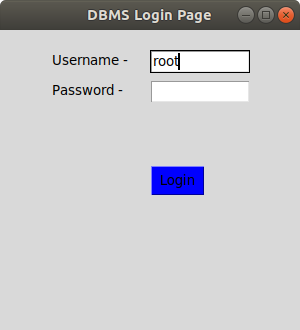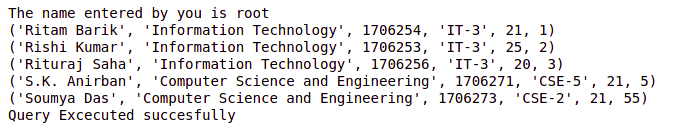# Create MySQL Database Login Page in Python using Tkinter

Prerequisites: Python GUI – tkinter, Python MySQL – Select Query

Tkinter is one of the Python libraries which contains many functions for the development of graphic user interface pages and windows. Login pages are important for the development of any kind of mobile or web application. This page is most essential for user authentication purposes.

We will use the `mysql.connector` library to establish a connection between Python project and MySQL workbench. Db is the object created using `mysql.connector.connect` class which stores all the information about databases such database name, password, and table name.

In the below example,

• `tk.label` and `tk.button` is used to create labels and buttons on the GUI screen. Every button contains a command in it which includes a function to be executed on click of the button.
• The function `logintodb` is created to login into the MySQL Database. The `savequery` includes the query to be executed on the click of the submit button.
• X and Y are the parameters given to adjust objects on the Tkinter window.
• Root.mainloop() is included at the last indicating that only components within it are included in the window.

Below is the implementation.

 `import` `tkinter as tk ` `import` `mysql.connector  ` `from` `tkinter ``import` `*`  `import` `PIL ` `  `  ` `  `def` `submitact(): ` `     `  `    ``user ``=` `Username.get() ` `    ``passw ``=` `password.get() ` `  `  `    ``print``(f``"The name entered by you is {user} {passw}"``) ` `  `  `    ``logintodb(user, passw) ` `  `  `  `  `def` `logintodb(user, passw): ` `     `  `    ``# If paswword is enetered by the  ` `    ``# user ` `    ``if` `passw: ` `        ``db ``=` `mysql.connector.connect(host ``=``"localhost"``, ` `                                     ``user ``=` `user, ` `                                     ``password ``=` `passw, ` `                                     ``db ``=``"College"``) ` `        ``cursor ``=` `db.cursor() ` `         `  `    ``# If no password is enetered by the ` `    ``# user ` `    ``else``: ` `        ``db ``=` `mysql.connector.connect(host ``=``"localhost"``, ` `                                     ``user ``=` `user, ` `                                     ``db ``=``"College"``) ` `        ``cursor ``=` `db.cursor() ` `         `  `    ``# A Table in the database ` `    ``savequery ``=` `"select * from STUDENT"` `     `  `    ``try``: ` `        ``cursor.execute(savequery) ` `        ``myresult ``=` `cursor.fetchall() ` `         `  `        ``# Printing the result of the ` `        ``# query ` `        ``for` `x ``in` `myresult: ` `            ``print``(x) ` `        ``print``(``"Query Excecuted succesfully"``) ` `         `  `    ``except``: ` `        ``db.rollback() ` `        ``print``(``"Error occured"``) ` `  `  `  `  `root ``=` `tk.Tk() ` `root.geometry(``"300x300"``) ` `root.title(``"DBMS Login Page"``) ` `  `  `C ``=` `Canvas(root, bg ``=``"blue"``, height ``=` `250``, width ``=` `300``) ` ` `  `# Definging the first row ` `lblfrstrow ``=` `tk.Label(root, text ``=``"Username -"``, ) ` `lblfrstrow.place(x ``=` `50``, y ``=` `20``) ` ` `  `Username ``=` `tk.Entry(root, width ``=` `35``) ` `Username.place(x ``=` `150``, y ``=` `20``, width ``=` `100``) ` `  `  `lblsecrow ``=` `tk.Label(root, text ``=``"Password -"``) ` `lblsecrow.place(x ``=` `50``, y ``=` `50``) ` ` `  `password ``=` `tk.Entry(root, width ``=` `35``) ` `password.place(x ``=` `150``, y ``=` `50``, width ``=` `100``) ` ` `  `submitbtn ``=` `tk.Button(root, text ``=``"Login"``,  ` `                      ``bg ``=``'blue'``, command ``=` `submitact) ` `submitbtn.place(x ``=` `150``, y ``=` `135``, width ``=` `55``) ` ` `  `root.mainloop() `

Output:My Personal Notes arrow_drop_upCheck out this Author's contributed articles.

If you like GeeksforGeeks and would like to contribute, you can also write an article using contribute.geeksforgeeks.org or mail your article to contribute@geeksforgeeks.org. See your article appearing on the GeeksforGeeks main page and help other Geeks.

Please Improve this article if you find anything incorrect by clicking on the "Improve Article" button below.

Article Tags :

Be the First to upvote.

Please write to us at contribute@geeksforgeeks.org to report any issue with the above content.